Courses

# Test: Resonance - 2

## 10 Questions MCQ Test Network Theory (Electric Circuits) | Test: Resonance - 2

Description
This mock test of Test: Resonance - 2 for Electrical Engineering (EE) helps you for every Electrical Engineering (EE) entrance exam. This contains 10 Multiple Choice Questions for Electrical Engineering (EE) Test: Resonance - 2 (mcq) to study with solutions a complete question bank. The solved questions answers in this Test: Resonance - 2 quiz give you a good mix of easy questions and tough questions. Electrical Engineering (EE) students definitely take this Test: Resonance - 2 exercise for a better result in the exam. You can find other Test: Resonance - 2 extra questions, long questions & short questions for Electrical Engineering (EE) on EduRev as well by searching above.
QUESTION: 1

### The maximum voltage across capacitor would be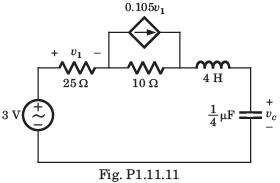Solution:

Thevenin equivalent seen by L - C combination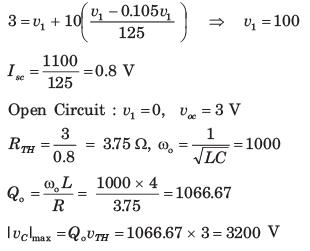QUESTION: 2

### For the circuit shown in fig. P1.1.11 resonant frequency fo is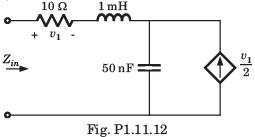Solution:

Applying 1 A at input port V1 = 10 V
voltage across 1 A source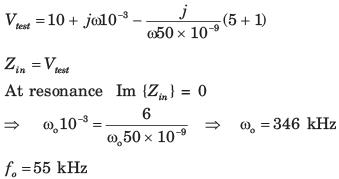QUESTION: 3

### For the circuit shown in fig. P1.11.13 the resonant frequency f0 is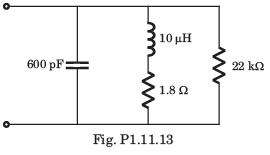Solution: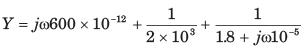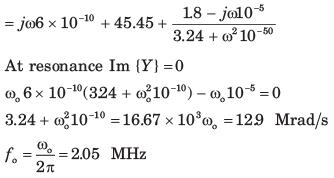QUESTION: 4

The network function of circuit shown in fig.P1.11.14 is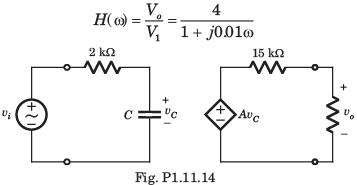The value of the C and A is

Solution: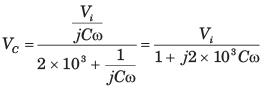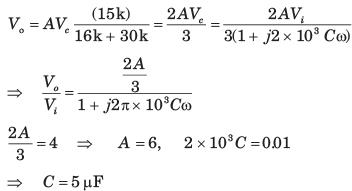QUESTION: 5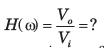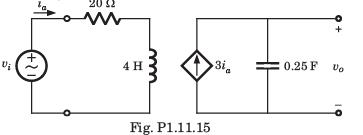Solution: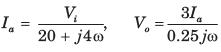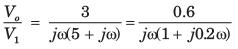QUESTION: 6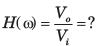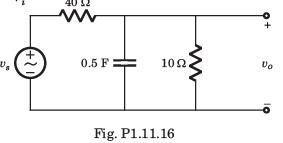Solution: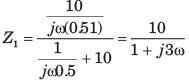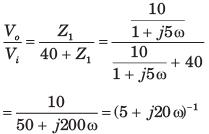QUESTION: 7

The value of input frequency is required to cause a gain equal to 1.5. The value is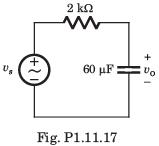Solution: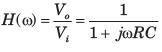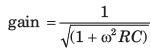For any value of ω, R,C gain ≤ 1.
Thus (D) is correct option.

QUESTION: 8

In the circuit of fig. P1.11.18 phase shift equal to -45is required at frequency ω = 20 rad/s . The value of R is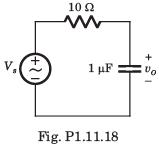Solution: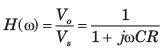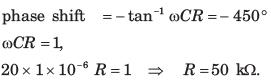QUESTION: 9

For the circuit of fig. P1.11.19 the input frequency is adjusted until the gain is equal to 0.6. The value of the frequency is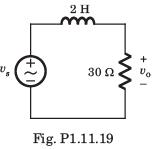Solution: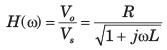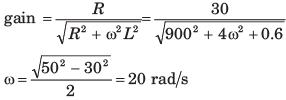QUESTION: 10

Bode diagram of the network function Vo/Vs for the circuit of fig. P1.11.30 is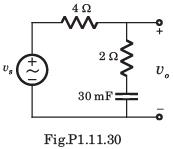Solution: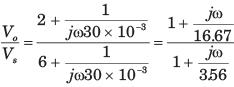-20 dB/decade line starting from ω = 5.56 rad s 20 dB/decade line starting from ω = 16.67 rad s Hence -20 dB/decade line for 5.56 < ω < 16.67 parallel to ω axis to ω > 16.67